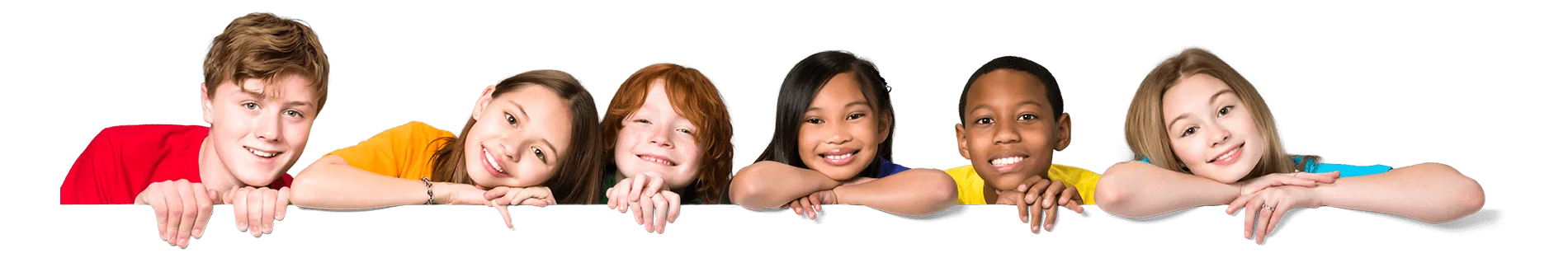Latest Results:

# 11plus Factors sample papers

Solve this Question

#### A 3-digit number 4a3 is added to another 3-digit number 984 to give a 4-digit number 13b7, which is divisible by 11. Then, (a + b) =?

 1. 10 2. 11 3. 12 4. 15

Solve this Question

#### The third multiple of 7 is

 1. 1 2. 7 3. 14 4. 21

Solve this Question

#### Find all the factors of the number: 48

 1. 1, 2, 3, 4, 6, 8, 9,12, 16, 24,48 2. 1, 2, 3, 4, 6, 8, 12, 16, 24,48 3. 1, 2, 3, 4, 6, 16, 24,48 4. None

Solve this Question

#### The sum of 12p3 and 1540 is 278q, which is divisible by 11.Then what is the value of p + q?

 1. 6 2. 11 3. 9 4. 7